Anúncio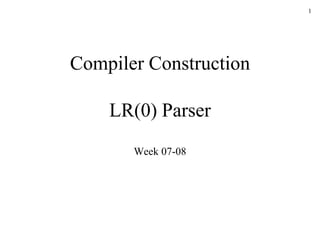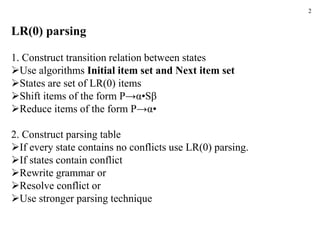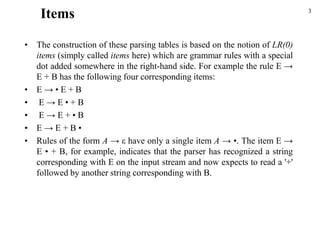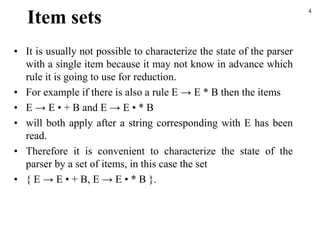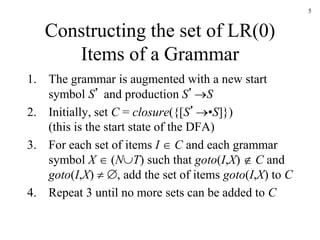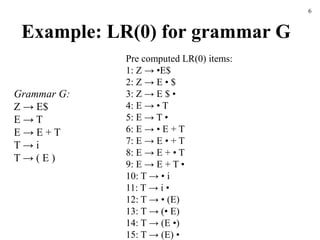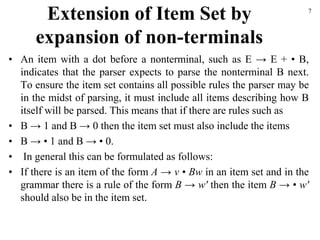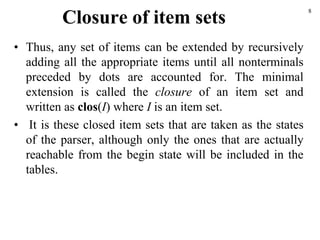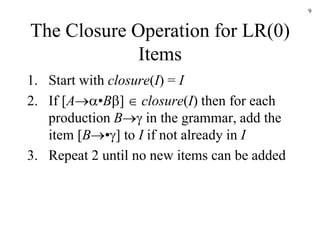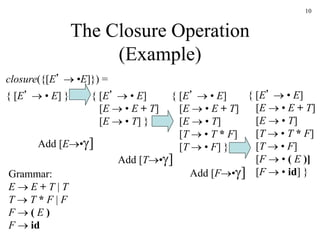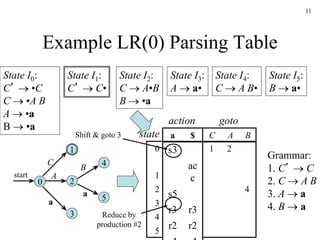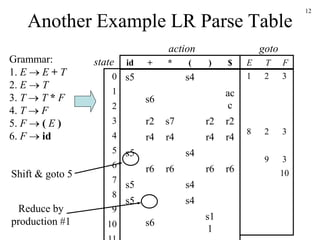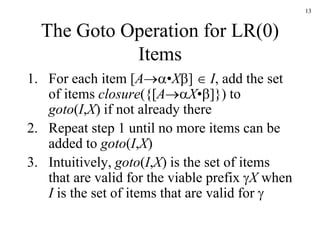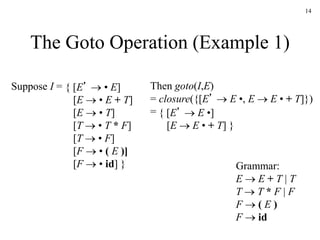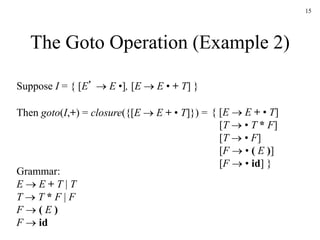1 de 15
Anúncio

### CC Week 07-08.ppt

1. 1 Compiler Construction LR(0) Parser Week 07-08
2. 2 LR(0) parsing 1. Construct transition relation between states Use algorithms Initial item set and Next item set States are set of LR(0) items Shift items of the form P→α•Sβ Reduce items of the form P→α• 2. Construct parsing table If every state contains no conflicts use LR(0) parsing. If states contain conflict Rewrite grammar or Resolve conflict or Use stronger parsing technique
3. • The construction of these parsing tables is based on the notion of LR(0) items (simply called items here) which are grammar rules with a special dot added somewhere in the right-hand side. For example the rule E → E + B has the following four corresponding items: • E → • E + B • E → E • + B • E → E + • B • E → E + B • • Rules of the form A → ε have only a single item A → •. The item E → E • + B, for example, indicates that the parser has recognized a string corresponding with E on the input stream and now expects to read a '+' followed by another string corresponding with B. 3 Items
4. Item sets • It is usually not possible to characterize the state of the parser with a single item because it may not know in advance which rule it is going to use for reduction. • For example if there is also a rule E → E * B then the items • E → E • + B and E → E • * B • will both apply after a string corresponding with E has been read. • Therefore it is convenient to characterize the state of the parser by a set of items, in this case the set • { E → E • + B, E → E • * B }. 4
5. 5 Constructing the set of LR(0) Items of a Grammar 1. The grammar is augmented with a new start symbol S’ and production S’S 2. Initially, set C = closure({[S’•S]}) (this is the start state of the DFA) 3. For each set of items I  C and each grammar symbol X  (NT) such that goto(I,X)  C and goto(I,X)  , add the set of items goto(I,X) to C 4. Repeat 3 until no more sets can be added to C
6. Example: LR(0) for grammar G 6 Grammar G: Z → E\$ E → T E → E + T T → i T → ( E ) Pre computed LR(0) items: 1: Z → •E\$ 2: Z → E • \$ 3: Z → E \$ • 4: E → • T 5: E → T • 6: E → • E + T 7: E → E • + T 8: E → E + • T 9: E → E + T • 10: T → • i 11: T → i • 12: T → • (E) 13: T → (• E) 14: T → (E •) 15: T → (E) •
7. Extension of Item Set by expansion of non-terminals • An item with a dot before a nonterminal, such as E → E + • B, indicates that the parser expects to parse the nonterminal B next. To ensure the item set contains all possible rules the parser may be in the midst of parsing, it must include all items describing how B itself will be parsed. This means that if there are rules such as • B → 1 and B → 0 then the item set must also include the items • B → • 1 and B → • 0. • In general this can be formulated as follows: • If there is an item of the form A → v • Bw in an item set and in the grammar there is a rule of the form B → w' then the item B → • w' should also be in the item set. 7
8. Closure of item sets • Thus, any set of items can be extended by recursively adding all the appropriate items until all nonterminals preceded by dots are accounted for. The minimal extension is called the closure of an item set and written as clos(I) where I is an item set. • It is these closed item sets that are taken as the states of the parser, although only the ones that are actually reachable from the begin state will be included in the tables. 8
9. 9 The Closure Operation for LR(0) Items 1. Start with closure(I) = I 2. If [A•B]  closure(I) then for each production B in the grammar, add the item [B•] to I if not already in I 3. Repeat 2 until no new items can be added
10. 10 The Closure Operation (Example) Grammar: E  E + T | T T  T * F | F F  ( E ) F  id { [E’  • E] } closure({[E’  •E]}) = { [E’  • E] [E  • E + T] [E  • T] } { [E’  • E] [E  • E + T] [E  • T] [T  • T * F] [T  • F] } { [E’  • E] [E  • E + T] [E  • T] [T  • T * F] [T  • F] [F  • ( E )] [F  • id] } Add [E•] Add [T•] Add [F•]
11. 11 Example LR(0) Parsing Table s3 ac c s5 r3 r3 r2 r2 a \$ 0 1 2 3 4 5 C A B 1 2 4 State I0: C’  •C C  •A B A  •a B  •a State I1: C’  C• State I2: C  A•B B  •a State I3: A  a• State I4: C  A B• State I5: B  a• 1 2 4 5 3 0 start a A C B a Grammar: 1. C’  C 2. C  A B 3. A  a 4. B  a action goto state Shift & goto 3 Reduce by production #2
12. 12 Another Example LR Parse Table Grammar: 1. E  E + T 2. E  T 3. T  T * F 4. T  F 5. F  ( E ) 6. F  id s5 s4 s6 ac c r2 s7 r2 r2 r4 r4 r4 r4 s5 s4 r6 r6 r6 r6 s5 s4 s5 s4 s6 s1 1 id + * ( ) \$ 0 1 2 3 4 5 6 7 8 9 10 E T F 1 2 3 8 2 3 9 3 10 Shift & goto 5 Reduce by production #1 action goto state
13. 13 The Goto Operation for LR(0) Items 1. For each item [A•X]  I, add the set of items closure({[AX•]}) to goto(I,X) if not already there 2. Repeat step 1 until no more items can be added to goto(I,X) 3. Intuitively, goto(I,X) is the set of items that are valid for the viable prefix X when I is the set of items that are valid for 
14. 14 The Goto Operation (Example 1) Suppose I = Then goto(I,E) = closure({[E’  E •, E  E • + T]}) = { [E’  E •] [E  E • + T] } Grammar: E  E + T | T T  T * F | F F  ( E ) F  id { [E’  • E] [E  • E + T] [E  • T] [T  • T * F] [T  • F] [F  • ( E )] [F  • id] }
15. 15 The Goto Operation (Example 2) Suppose I = { [E’  E •], [E  E • + T] } Then goto(I,+) = closure({[E  E + • T]}) = { [E  E + • T] [T  • T * F] [T  • F] [F  • ( E )] [F  • id] } Grammar: E  E + T | T T  T * F | F F  ( E ) F  id
Anúncio